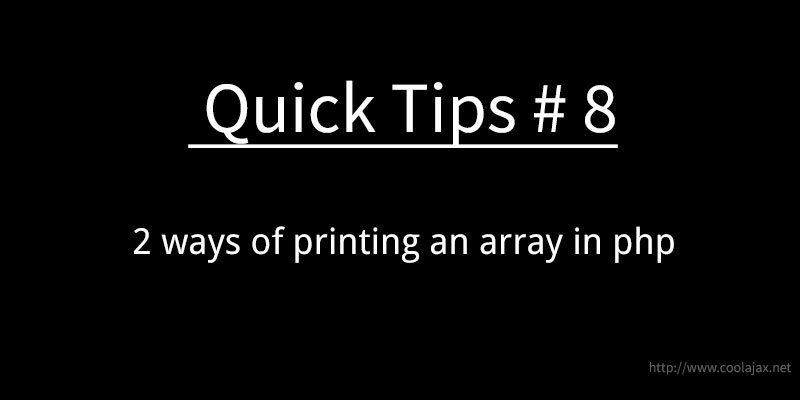# 2 ways of printing an array in phpPHP provides two very useful functions that can be used to output a variable’s value recursively called print_r() and var_dump().

#### – Create An Array:

`\$my_array = array("elem_1","elem_2","elem_3");`

#### – Using Print_r() function:

Print_r() can return its output as a string, as opposed to writing it to the scripts standard output

```print_r(\$my_array);

// Output:
// Array (  => elem_1  => elem_2  => elem_3 )```

#### – Using var_dump() function:

Var_dump() is capable of outputting the value of more than one variable at the same time. Var_dump() outputs the data types of each value.

```var_dump(\$my_array);

// Output:
// array(3) { => string(6) "elem_1" => string(6) "elem_2" => string(6) "elem_3" }```